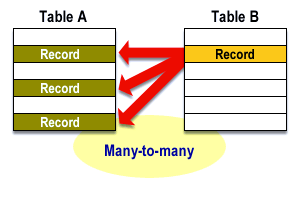ER Diagrams   «Prev  Next»
 Lesson 8 Many-to-many Relationships, part 1 Objective Define many-to-many Relationships.

# Define Many-to-many Relationships

A many-to-many (M:N) relationship exists when, for one instance of entity A, there exists zero, one, or many instances of entity B; and for one instance of entity B, there exists zero, one, or many instances of entity A.
To illustrate such a relationship graphically, think of entities A and B in terms of generic tables. A many-to-many relationship exists when one record in Table A can be associated with many records in Table B; and one record in Table B can be associated with many records in Table A. The following diagrams illustrates an M:N relationship between Tables A and B from the viewpoint first of Table A, then of Table B.1) The diagram above illustrates an M:N relationship between Table A and B from the viewpoint of Table A. Note that the record in Table A is related to many records in Table B (A:B = 1:N).2) The relationship between Tables A and B from the viewpoint of Table B appears this way. Note that one record in Table B is related to many records in Table A (B:A = 1:M).
1. The diagram above illustrates an M:N relationship between Table A and B from the viewpoint of Table A
2. The relationship between Tables A and B from the viewpoint of Table B appears this way.

## Many-to-many Relationship in Database

Many to many relationships can only exist while you conceptually design the database and this is when you need to eliminate them. You physically cannot have it in a working database otherwise your schema would need to change continually.
A many-to-many (M:N) relationship, sometimes called non-specific, is when for one instance of entity A, there are zero, one, or many instances of entity B and for one instance of entity B there are zero, one, or many instances of entity A.
An example is:
"employees can be assigned to no more than two projects at the same time; projects must have assigned at least three employees"

## Dealing with Many-to-Many Relationships

As you read earlier, there are problems with many-to-many relationships.
1) First Problem: The relational data model cannot handle many-to-many relationships directly and is limited to one-to-one and one-to-many relationships. This means that you must replace the many-to-many relationships that you have identified in your database environment with a collection of one-to-many relationships if you want to be able to use a relational DBMS.
2) Second problem: To understand this problem, consider the relationship between an order a customer places with DistributedNetworks and the merchandise items on the order. There is a many-to-many relationship between the order and the item because each order can be for many items and each item can appear on many orders (typically orders from different customers). Whenever a customer orders an item, the number of copies of the item varies, depending on how many copies the customer needs.
Yes, typically people order only one copy of a movie, but we need to allow them to order as many as they want.
Question: Where should we store the quantity being ordered?
It cannot be part of the order entity because the quantity depends on which item we are talking about. By the same token, the quantity cannot be part of the item entity because the quantity depends on the specific order. This scenario is known as relationship data, which is data that applies to the relationship between two entities rather than to the entities themselves. Relationships, however, cannot have attributes. We therefore must have some entity to represent the relationship between the two, an entity to which the relationship data can belong.

The next lesson explores many-to-many relationships further.